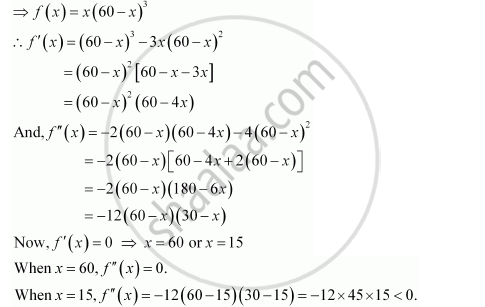Share

# Find Two Positive Numbers X and Y Such that X + Y = 60 and Xy3 is Maximum. - CBSE (Commerce) Class 12 - Mathematics

#### Question

Find two positive numbers x and y such that x + y = 60 and xy3 is maximum.

#### Solution

The two numbers are x and y such that x + y = 60.

⇒ y = 60 − x

Let f(x) = xy3.∴By second derivative test, = 15 is a point of local maxima of f. Thus, function xy3 is maximum when x = 15 and y = 60 − 15 = 45.

Hence, the required numbers are 15 and 45.

Is there an error in this question or solution?

#### APPEARS IN

NCERT Solution for Mathematics Textbook for Class 12 (2018 to Current)
Chapter 6: Application of Derivatives
Q: 14 | Page no. 233

#### Video TutorialsVIEW ALL 

Solution Find Two Positive Numbers X and Y Such that X + Y = 60 and Xy3 is Maximum. Concept: Maxima and Minima.
S